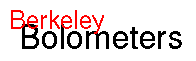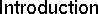email: jmg@physics.berkeley.edu

# Why bolometers?

Observations in the far-IR to mm wavelength region are opening a new window on the universe. For example, recent measurements of the cosmic microwave background anisotropy by BOOMERanG [debernardis00] and MAXIMA [hanany00] lend strong support to inflationary cosmological models with a geometry close to flat. A new population of dusty luminous objects that may account for a significant fraction of all star formation is being explored by ground-based telescopes such as SCUBA/JCMT. Both of these types of observation have been possible only because of large improvements in the sensitivity of bolometric receivers. In the future, further large improvements in sensitivity will be possible by increasing the size of bolometer arrays. The Voltage-Biased Superconducting Bolometer (VSB) is a good candidate for large bolometer arrays because the sensors can be made by standard optical lithography and the large noise margin of the VSB-SQUID combination enables readout multiplexing.

# What is a bolometer?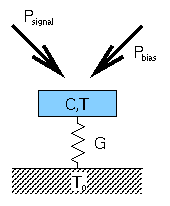A bolometer (or calorimeter) is a detector for radiation or particles. We use bolometers to detect light in the far-infrared and mm-waves. These detectors typically function as follows: An absorber of heat capacity C is thermally connected to a heat reservoir at temperature T0 by a weak thermal link G. The absorber sees the power of the incoming light Psignal and an electrical bias power Pbias and hence has a temperature T=T0+ (Psignal+Pbias)/G>T0. If the incoming power Psignal changes and Pbias stays constant the temperature T will change. A bolometer works by measuring this change of T with a thermometer which is directly attached to the absorber.

As a thermometer one commonly uses a material which changes strongly in resistivity with temperature in the regime of interest. An example of such a material are neutron-transmutation doped Ge-crystals (NTDs). The resistance R of a crystal is measured by biasing the thermometer with a constant current I and measuring the change in Voltage V. The electrical bias power then is Pbias=IV=V2/R=I2R.

The thermal time constant of the detector is given by the ratio of the heat capacity and the thermal cunductance: t0=C/G.

# Why superconducting?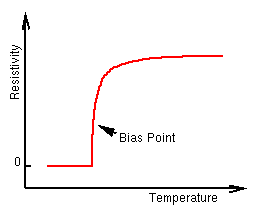In the resistive transition superconductors show the strongest known dependence of resistivity on temperature. If one can manage to hold a superconductor right in its transition a small change in temperature will lead to a large change in resistance which can be measured accurately. A superconductor is hence an ideal candidate for a thermometer in a bolometer.

# Why voltage-biased?

To measure the resistance of the superconducting thermometer one has two choices:

current-biased mode
The thermometer is biased with a constant current I0 and the resistance is measured by measuring the voltage. The electrical power in the bolometer will be Pbias=I02R.
voltage-biased mode
The thermometer is biased with a constant voltage V0 and the resistance is measured by measuring the current. The electrical power in the bolometer will be Pbias=V02/R.
The current-biased mode has the advantage that a voltage can be amplified relatively easily. However, with the arrival of SQUIDS (superconducting quantum interference devices) an adequate amplification and detection of a current signal has become possible. This makes it possible to run the superconducting bolometer in the voltage-biased mode.

If the thermometer is voltage-biased the electrical power is given by Pbias=V02/R. Then an increase of the incoming signal power Psignal (which tends to increase T and therefore R) will lead to a decrease in Pbias. If the thermometer is biased in the steep part of its transition the total power on the bolometer Ptotal=Psignal+Pbias and therefore the temperature T will essentially remain constant. This effect is referred to as "strong negative electrothermal feedback". It has several important advantages:

• It speeds up the detector. Theory and experiment show that in this mode the detector-time-constant can be more then two orders of magnitude smaller than the thermal time constant C/G.
• The detector is linear over a wide range of incoming signal power, i.e. the ratio of detector-signal to the incoming light-signal is a constant. This will greatly simplify the use of such bolometers.
• Because of the negative feedback the thermometer stays within its transition by itself once the correct bias-voltage is applied. No external feedback circuit is necessary.

# Why an absorber mesh?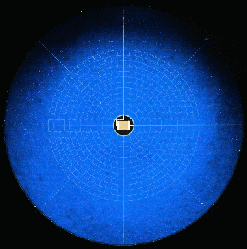The spider web in this picture consists of 1µm thick silicon nitride. The superconducting thermometer is visible in the center. The absorbing mesh around it is 3.5mm in diameter. It is suspended by 1mm long legs which are 5µm wide. With this suspension technique very low values of G (down to 6*10-12W/K) can be achieved, suitable for low power applications like the measurement of the cosmic background anisotropy.

The structure of the mesh is much smaller than the wavelength of the radiation to be detected (~ 1mm) and the incoming light is absorbed efficiently. At the same time cosmic rays will only be absorbed if they actually hit one of the segments of the mesh. Since the filling factor of the spider web is < 10% most cosmic rays will pass through. This is important to reduce detector dead time.